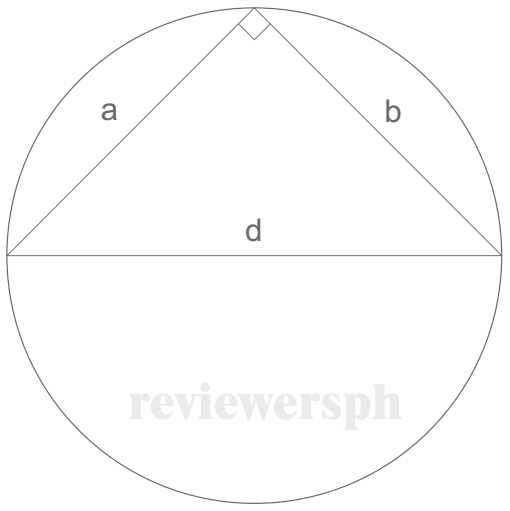### Math Notes

Subjects

#### Plane Geometry Solutions

##### Topics || Problems

Calculate the area of the circle circumscribing an isosceles right triangle having an area of 242 mm2.Since the triangle is a right triangle, one side must be the diameter of the circle. Thus,

$$A_{tri} = \frac{1}{2}ab$$

But a = b

$$A_{tri} = \frac{1}{2}a^2$$

$$242 = \frac{1}{2}a^2$$

$$a = 22 mm$$

$$a^2 +a^2 = d^2$$

$$d = 22\sqrt{2}$$

$$A_{circle} = \frac{ \pi d^2}{4}$$

$$A_{circle} = \frac{ \pi (22\sqrt{2})^2}{4}$$

$$A_{circle} = 760.27 mm^2$$